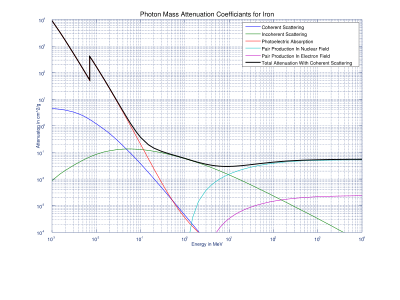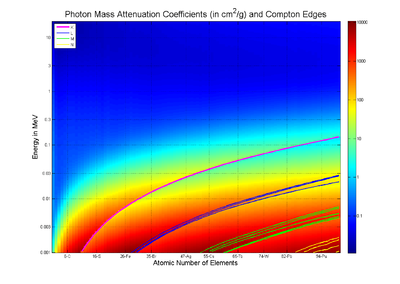# Mass attenuation coefficient

Mass attenuation coefficient

The mass attenuation coefficient is a measurement of how strongly a chemical species or substance absorbs or scatters light at a given wavelength, per unit mass. In addition to visible light, mass attenuation coefficients can be defined for other electromagnetic radiation (such as X-rays), sound, or any other beam that attenuates.

The mass attenuation coefficient is also called mass absorption coefficient or mass extinction coefficient. Although all three terms are usually used interchangeably, they can occasionally have a subtle distinction, as explained below.

The mass extinction coefficient should not be confused with the different definition of "extinction coefficient" used more commonly in physics, namely the imaginary part of the complex index of refraction (which is unitless). In fact, they have a straightforward but nontrivial relationship; see Mathematical descriptions of opacity.

## Defining equations

The defining equation for the mass attenuation coefficient is essentially a different way to write the Beer-Lambert law. The Beer-Lambert law is normally written$I=I_0 \, e^{-\mu \ell}$

where

I0 is the original intensity of the beam,
I is the intensity of the beam at distance$\ell$ into the substance,
e is Euler's number, about 2.718,
μ is the attenuation coefficient.

When discussing the mass attenuation coefficient, this equation is rewritten$I = I_0 \, e^{-(\mu/\rho)\rho \ell}$

where

ρ is the density, (μ / ρ) is the mass attenuation coefficient and$\rho \ell$ is the area density known also as mass thickness.

In accordance with the above, the mass attenuation coefficient is equal to the attenuation coefficient divided by the density.

### In solutions

In chemistry, mass attenuation coefficients are often used for a chemical species dissolved in a solution. In that case, the mass attenuation coefficient is defined by the same equation, except that the "density" is the density of only that one chemical species, and the "attenuation" is the attenuation due to only that one chemical species. The actual attenuation coefficient is computed by$\mu = (\mu/\rho)_1 \rho_1 + (\mu/\rho)_2 \rho_2 + \cdots$

where each term in the sum is the mass attenuation coefficient and density of a different component of the solution (the solvent must also be included). This is a convenient concept because the mass attenuation coefficient of a species is approximately independent of its concentration (as long as certain assumptions are fulfilled).

A closely related concept is molar absorptivity. They are quantitatively related by:

(Mass attenuation coefficient)×(Molar mass) = (Molar absorptivity).

### Units

The attenuation coefficient has units of inverse-length, while density has units of mass per volume. Since the mass attenuation coefficient is the ratio of these two, we find that it has units of (length-squared) per mass. The SI unit is m2kg-1. Other common units include cm2g-1 (the most common unit for X-ray mass attenuation coefficients) and mL×g-1cm-1 (sometimes used in solution chemistry).

## Mass attenuation coefficients for X-raysMass Attenuation Coefficient of Iron with contributing sources of attenuation: Coherent scattering, Incoherent scattering, Photoelectric Absorption, and two types of Pair Production. The discontinuity of Photoelectric Absorption values are due to K-edge. Graph data came from NIST's XCOM database.Mass Attenuation Coefficient values shown for all elements with atomic number Z smaller than 100 collected for photons with energies from 1 keV to 20 MeV. The discontinuities in the values are due to absorption edges which were also shown.

Tables of photon mass attenuation coefficients are essential in radiological physics, radiography (for medical and security purposes), dosimetry, diffraction, interferometry, crystallography and other branches of physics. The photons can be in form of x-ray, gamma-ray, and bremsstrahlung.

The values of mass attenuation coefficients are dependent upon the absorption and scattering of the incident radiation caused by several different mechanisms such as:

The actual values have been thoroughly examined and are available to the general public through three databases run by National Institute of Standards and Technology (NIST):

1. XAAMDI database
2. XCOM database
3. FFAST database

## Calculating the composition of a solution

If several known chemicals are dissolved in a single solution, the concentrations of each can be calculated using a light absorption analysis. First, the mass attenuation coefficients of each individual solute or solvent, ideally across a broad spectrum of wavelengths, must be measured or looked up. Second, the attenuation coefficient of the actual solution must be measured. Finally, using the formula$\mu = (\mu/\rho)_1 \rho_1 + (\mu/\rho)_2 \rho_2 + \cdots$

the spectrum can be fitted using$\rho_1,\rho_2,\ldots$ as adjustable parameters. (Remember, μ and each (μ / ρ)i are functions of wavelength.)

If there are N solutes or solvents, this procedure requires at least N measured wavelengths to create a solvable system of simultaneous equations, although using more wavelengths gives more reliable data.

## Attenuation versus absorption

The terms "mass attenuation coefficient", "mass absorption coefficient", and "mass extinction coefficient" are almost always used interchangeably. However, in certain situations they are distinguished, as follows.

When a narrow (collimated) beam of light passes through a substance, the beam will lose intensity due to two processes: The light can be absorbed by the substance, or the light can be scattered (i.e., the photons can change direction) by the substance. Just looking at the narrow beam itself, the two processes cannot be distinguished. However, if you set up a detector to measure light leaving in different directions, or conversely try using a non-narrow beam, you can measure how much of the lost intensity was scattered, and how much was absorbed.

In this context, the "mass absorption coefficient" measures how quickly the beam would lose intensity due to the absorption alone, while "mass attenuation coeffient" measures the total loss of narrow-beam intensity, including scattering as well. The "mass extinction coefficient" can be either.

## References and footnotes

1. ^ Hubbell, J. H.; Seltzer, S. M.. "Tables of X-Ray Mass Attenuation Coefficients and Mass Energy-Absorption Coefficients". National Institute of Standards and Technology (NIST). Retrieved September 2007.
2. ^ Berger, M.J.; J.H. Hubbell, S.M. Seltzer, J. Chang, J.S. Coursey, R. Sukumar, and D.S. Zucker. "XCOM: Photon Cross Sections Database". National Institute of Standards and Technology (NIST). Retrieved September 2007.
3. ^ Chantler, C.T.; Olsen, K., Dragoset, R.A., Chang, J., Kishore, A.R., Kotochigova, S.A., and Zucker, D.S.. "X-Ray Form Factor, Attenuation and Scattering Tables (version 2.1).". National Institute of Standards and Technology (NIST). Retrieved September 2007.

Wikimedia Foundation. 2010.

Поможем написать реферат

### Look at other dictionaries:

• mass attenuation coefficient — masinis silpninimo koeficientas statusas T sritis fizika atitikmenys: angl. mass attenuation coefficient vok. Massenschwächungskoeffizient, m rus. массовый коэффициент ослабления, m pranc. coefficient d’atténuation massique, m; coefficient… …   Fizikos terminų žodynas

• mass attenuation coefficient — masinis silpimo koeficientas statusas T sritis Standartizacija ir metrologija apibrėžtis Apibrėžtį žr. priede. priedas( ai) Grafinis formatas atitikmenys: angl. mass attenuation coefficient vok. Massenschwächungskoeffizient, m rus. массовый… …   Penkiakalbis aiškinamasis metrologijos terminų žodynas

• mass attenuation coefficient — masinis silpimo koeficientas statusas T sritis Standartizacija ir metrologija apibrėžtis Neelektringųjų jonizuojančiųjų dalelių atveju masinis medžiagos silpimo koeficientas μ /ρ išreiškiamas dN/N ir ρdl dalmeniu; dN/N – dalelių dalis, kuri… …   Penkiakalbis aiškinamasis metrologijos terminų žodynas

• mass attenuation coefficient — the linear attenuation coefficient divided by the density of the absorbing material …   Medical dictionary

• Attenuation coefficient — For attenuation coefficient as it applies to electromagnetic theory and telecommunications see propagation constant. For the mass attenuation coefficient , see the article mass attenuation coefficient. The attenuation coefficient is a quantity… …   Wikipedia

• coefficient d’atténuation massique — masinis silpninimo koeficientas statusas T sritis fizika atitikmenys: angl. mass attenuation coefficient vok. Massenschwächungskoeffizient, m rus. массовый коэффициент ослабления, m pranc. coefficient d’atténuation massique, m; coefficient… …   Fizikos terminų žodynas

• coefficient d’atténuation par unité de masse surfa — masinis silpninimo koeficientas statusas T sritis fizika atitikmenys: angl. mass attenuation coefficient vok. Massenschwächungskoeffizient, m rus. массовый коэффициент ослабления, m pranc. coefficient d’atténuation massique, m; coefficient… …   Fizikos terminų žodynas

• coefficient d’atténuation massique — masinis silpimo koeficientas statusas T sritis Standartizacija ir metrologija apibrėžtis Apibrėžtį žr. priede. priedas( ai) Grafinis formatas atitikmenys: angl. mass attenuation coefficient vok. Massenschwächungskoeffizient, m rus. массовый… …   Penkiakalbis aiškinamasis metrologijos terminų žodynas

• coefficient d’atténuation massique — masinis silpimo koeficientas statusas T sritis Standartizacija ir metrologija apibrėžtis Neelektringųjų jonizuojančiųjų dalelių atveju masinis medžiagos silpimo koeficientas μ /ρ išreiškiamas dN/N ir ρdl dalmeniu; dN/N – dalelių dalis, kuri… …   Penkiakalbis aiškinamasis metrologijos terminų žodynas

• coefficient — 1. The expression of the amount or degree of any quality possessed by a substance, or of the degree of physical or chemical change normally occurring in that substance under stated conditions. 2. The ratio or factor that relates a quantity… …   Medical dictionary